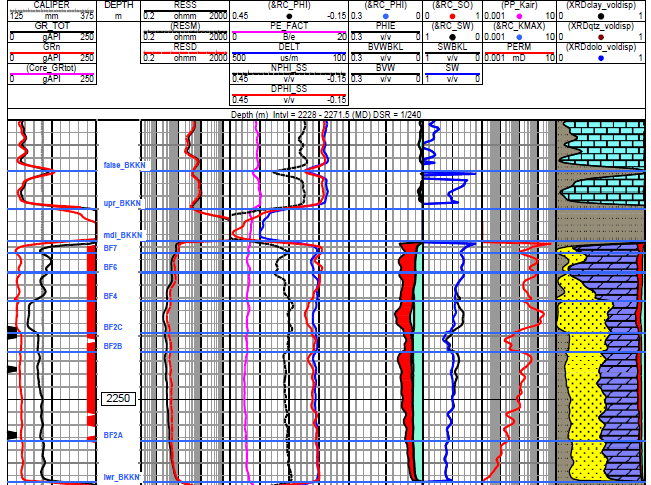LOG NORMALIZATION BASICS
Log normalization is based on the concept that maximum and minimum log readings in a zone in an area should have the same log reading. The assumption includes the fact that there are no major geological reasons for the values to vary across space.

Reasons for log normalization are numerous:  poor tool calibration, mis-labeled scales, unconventional units of measurement, mud weight, borehole size, temperature, rock alteration, and many more.

Log normalization is the process of re-scaling a log so that it matches its neighbours, based on some logical reasoning. Log normalization involves a "stretch" or "squeeze" of the data values between two scale end points or between two arbitrary log values. The math is shown in a later Section on this webpage.

Log re-scaling is a simplified form of normalization. It can involve an equal linear shift of the two scale end-points, or applying a multiplication factor to all data points.in the log curve. This is often needed to change older log curve scales to match modern logs or to convert Metric scales to English units and vice-versa.

For example,convert gama ray in units of ug-ra-equiv/ton to API units:
1:  GRapi = GRug-ra * 10

Convert neutron porosity from limestone to sandstone units:
2: PHIN_SS = PHIN_LS + 0.03

Convert somic log from English to Metric units:
3: DTCmetric = DTCenglish * 3.281

Re-scaling a resistivity log is a little trickier since the tools actually measures comductivity, so we need to create a COND curve from RESD:
4: COND = 1000 / RESD
Adlust the somde error by adding or subtracting a few milliSermens from COND. This will stretch or squeeze the high resistivity and do almost nothing to the low resistivity.

5: CONDnew = COND + X    where X = sonde error correction
Convert COND back to RESD:
6: RESDnew = 1000 / CONDnew

Log normalization is most often applied to gamma ray logs. Here we re-scale the GR logs so that a particular clean sand in all wells have the same GR value. Likewise, the key shale zones in all wells have the same GR value. This makes correlation of zones easier and helps the analyst to better understand reservoir quality without additional calculations.

The technique is widely used for sonic, density, density porosity, and neutron logs. In these cases, the equation is the same but the MIN and MAX terms apply to low and high log readings chosen in particular geological horizons, for example in a low porosity and a shale interval.

Another approach is to run a statistical analysis over the same geological interval in many wells, select the wells with similar min, max, and median values as "key" wells, then shift the outlier logs so that the median matches the key wells.CAUTION: Normalization can remove natural geological variations that may have significance in understanding the reservoir variations across space. For example, if calcite cement varies from place to place, normalization will remove porosity trends that vary with cementation. Similarly, on a GR log if feldspar content or clay type varies, this knowledge will be lost.NORMALIZATION WITH CONSTANT OFFSET
The equation is:
1: XXXn = XXX + SHIFT

Where:
XXXn =  normalized XXX log value
XXX = input XXX log curve value
SHIFT =  value that moves the median curve value in this zone in this well to the median value
of the key wells.COMMENTS
This method is helpful when neutron log scale (Sandstone vs Limestone) is unknown or ambiguous. Can be applied to any porosity log or the logarithm of resistivity. Not recommended for GR or SP..Numerical Example
Median sonic log value over selected interval in Key wells is DTCavg = 225 usec/m.
Median sonic log value over selected interval in THIS well is DTCavg = 221 usec/m.
SHIFT = 225 - 221 = +4 usec/m.
DTCn = DTC + 4NORMALIZATION By STREtCH or SQUEEZE
The equation is:
1: XXXn = XXXMIN + (XXXMAX - XXXMIN) * (XXX - XXXLOW) / (XXXHIGH - XXXLOW)

Where:
XXXn =  normalized log value
XXX = input log curve value
XXXMIN = minimum XXX log value to normalize to
XXXMAX = maximum XXX log value to normalize to
XXXLOW = actual XXX log value in this zone in this well that corresponds to XXXMIN
XXXHIGH = actual XXX log value in this zone in this well that corresponds to XXXMAXCOMMENTS
This is the method most used for GR and SP curves but can be used with care on any log curve.Nunerucak Example
Assume you want to re-scale all GR logs so that all clean lines are at 20 API units and all shale lines are at 120 API units. That makes GRMIN = 20 API units, GRMAX = 120 API units for all wells
Assume GRLOW = GR0 = 30 API units, GRHIGH = GR100 = 155 API units in THIS zone in this well.
Assume actual GR at a depth level = 55 API units
1: GRn = 20 + (120 - 20) * (55 - 30) / (155 - 30) = 40 API unitsRed curve in Track 1 is normalized GR (GRn), black curve is original gamma ray in a radioactive sand. Because uranium content is nearly constant, we can re-scale the total GR to a more useful shale indicator by normalizing. Parameters used were GRMIN = 25, GRMAX = 275, GRLO = GR0 = 100, GRHIGH = GR100 = 350
(off scale but available from LAS file).

Page Views ---- Since 01 Jan 2015
Copyright 2023 by Accessible Petrophysics Ltd.
CPH Logo, "CPH", "CPH Gold Member", "CPH Platinum Member", "Crain's Rules", "Meta/Log", "Computer-Ready-Math", "Petro/Fusion Scripts" are Trademarks of the Author